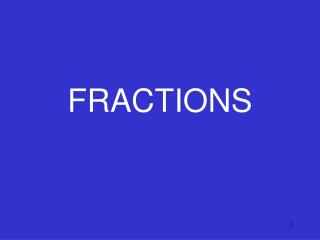DownloadDownload PresentationFRACTIONS

# FRACTIONS

Download Presentation## FRACTIONS

- - - - - - - - - - - - - - - - - - - - - - - - - - - E N D - - - - - - - - - - - - - - - - - - - - - - - - - - -
##### Presentation Transcript

1. FRACTIONS

2. VOCABULARY • Fraction-a number that describes part of a whole or part of a set. • Numerator-top number of a fraction that tells how many parts of the whole or set you have.

3. VOCABULARY • Denominator- bottom number of a fraction that tells how many parts there are in the whole or set. • Proper fraction-little number on top(numerator); big number on bottom(denominator)

4. VOCABULARY • Improper fraction– a fraction with a value greater than or equal to 1. • Mixed number- a whole number and a fraction together.

5. numerator denominator 5 parts shaded 8 parts in the whole

6. PROPER FRACTIONS small large

7. IMPROPER FRACTIONS large small

8. MIXED NUMBERS fraction whole number

9. Improper Fractions & Mixed Numbers

10. Mixed Numbers

11. Improper Fractions

12. To express amixed numberas animproper fraction,first multiply the whole number by thedenominator and add the numerator. Then write this sum over the denominator.

13. 2 2x2+ 1 = = 2 2 2

14. 2

15. To express animproper fractionas amixed number, divide the denominator into the numerator.

16. 2 2 3 2 = 3 3 -6 2

17. Express the mixed number as an improper fraction. 3 x 5 + 1 = = 5

18. Express the mixed number as an improper fraction. 5 x 9 + 8 = = 9

19. Express the improper fraction as a whole number. 4 4 = = -32 0